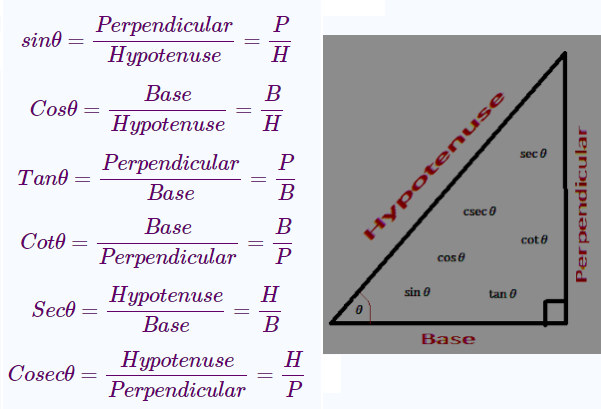### Dictionary Rank of a Word | Permutations & Combinations

PERMUTATIONS & COMBINATIONS Rank of the word or Dictionary order of the English words like COMPUTER, COLLEGE, SUCCESS, SOCCER, RAIN, FATHER, etc. Dictionary Rank of a Word Method of finding the Rank (Dictionary Order) of the word  “R A I N” Given word: R A I N Total letters = 4 Letters in alphabetical order: A, I, N, R No. of words formed starting with A = 3! = 6 No. of words formed starting with I = 3! = 6 No. of words formed starting with N = 3! = 6 After N there is R which is required R ----- Required A ---- Required I ---- Required N ---- Required RAIN ----- 1 word   RANK OF THE WORD “R A I N” A….. = 3! = 6 I……. = 3! = 6 N….. = 3! = 6 R…A…I…N = 1 word 6 6 6 1 TOTAL 19 Rank of “R A I N” is 19 Method of finding the Rank (Dictionary Order) of the word  “F A T H E R” Given word is :  "F A T H E R" In alphabetical order: A, E, F, H, R, T Words beginni

### Lesson Plan Math Class X (Ch-8) | Trigonometry

E- LESSON PLAN   SUBJECT MATHEMATICS    CLASS 10
lesson plan for maths class 10 chapter 8 & 9 Trigonometry and application of trigonometry, lesson plans for mathematics teachers,  Method to write lesson plan for maths class 10,  lesson plan for maths in B.Ed.

 Board - CBSE CLASS –X SUBJECT- MATHEMATICS CHAPTERS  8 & 9  :- Trigonometry and applications of trigonometry

TOPIC:-

Chapter 8 & 9 Trigonometry and Application of Trigonometry

DURATION:-

These topics are divided into seven modules and are completed in ten class meetings.

PRE- REQUISITE KNOWLEDGE:-
TEACHING AIDS:-

Green Board, Chalk,  Duster, Charts, smart board, laptop, projector  etc.

METHODOLOGY:-  Demonstration and Lecture method.

OBJECTIVES:-
• Introduction, and basic formulas of trigonometry.
• Problems based on basic formulas.
• Values of trigonometric ratios on standard angles 0o,30o, 45o, 60o, 90o
• Trigonometric transformations in first quadrant.
• Trigonometric identities and their applications in different problems.
• Applications of trigonometry in day to day life (Heights and distances).

Lesson Plan For CBSE Class 10 (Chapter 8) For Mathematics Teacher

PROCEDURE :-

Start the session by checking their previous knowledge, by asking the questions related to the right angled triangle, Pythagoras theorem and algebraic identities. After this explain the topic to the students.

 S.NO. TOPIC 1 Introduction Teacher will start the session by asking some questions about different types of triangles, then explain the properties of right angled triangle and the Pythagoras theorem. (Hypotenuse)2 = (Base)2 + (Perpendicular)2 Now teacher will introduce the topic Trigonometry. Trigonometry is the branch of mathematics dealing with the relations of the sides and angles of triangle. Trigonometry is the word made up of two Greek words, Trigonon and metron. Trigonon means triangle and metron means to measure. So trigonometry means to measure the sides and angles of a triangle. In trigonometry there are six functions of angles, they are named as  sine (sin), cosine (cos), tangent (tan), cotangent (cot), secant (sec), cosecant (cosec). 2 Trigonometric ratios Now teacher will explain the relationship between the six trigonometric functions, angles and sides of a right angled triangleTeacher will also explain all these relations with the help of some problems. 3 TRIGONOMETRIC FUNCTIONS WITH STANDARD ANGLENow teacher will explain the method of finding the values of trigonometric functions with the standard angle (0o,  30o,  45o, 60o, 90o) and explain to the students , the implementation of these formulas in different problems. Teacher will also provide sufficient problems to the students for practice. 4 TRANSFORMATION OF TRIGONOMETRIC FUNCTIONS  Now teacher will explain the transformations of trigonometric functions as follows  sin(90 - θ)   = cosθ,                        cos(90 - θ) =  sinθ, tan(90 - θ)  = cotθ,                          cot(90 - θ) = tanθ, sec(90 - θ)   = cosecθ,                  cosec(90 - θ) = secθ Teacher will also explain the implementation of these ratios in different problems 5 INTRODUCTION OF TRIGONOMETRIC IDENTITIES Now teacher will explain the method of finding the trigonometric identities and will also solve some questions on the board so that students become familiar with the method of implementation of these identitiesTeacher will also assign some problems to the students for practice. 6 APPLICATIONS OF TRIGONOMETRY Now teacher will explain the different situations in which trigonometry can be implemented. In order to cover this topic teacher will explain the Angle of Elevation, Angle of Depression and explain these by apply trigonometry in simple and daily life problems. 7 Now teacher will explain the Application of trigonometry in the problems like heights and distances or on complex daily life problems.

EXPECTED OUTCOMES:-
After studying this lesson students should know
• All six types of trigonometric functions.
• Relationships between trigonometric functions, angles and sides.
• Values of trigonometric functions with standard angles.
• Transformations of trigonometric functions.
• Trigonometric Identities and their Implementations.
• Method of solving the problems with the help of trigonometry.
STUDENTS DELIVERABLES:-
• Review questions given by the teacher.
• Students should prepare the presentation on the trigonometric identities.
• Solve N.C.E.R.T. problems with examples.
• Solve assignment given by the teacher.
EXTENDED LEARNING:-

Students can extend their learning through the RESOURCE CENTRE and can find more valuable and interesting concepts on mathematics at  cbsemathematics.com

ASSESSMENT TECHNIQUES:-
Assignment sheet will be given as home work at the end of the topic. Separate sheets which will include questions of logical thinking and Higher order thinking skills will be given to the above average students.
Class Test , Oral Test , worksheet , Assignments(For Chapter 8) and Assignment (For Chapter 9) can be made the part of assessment.
Re-test(s) will be conducted on the basis of the performance of the students in the test.

THANKS FOR YOUR VISIT